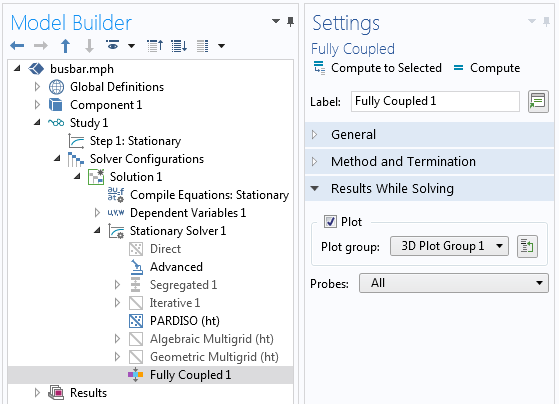## 解决方法

#### 载荷缓慢变化“辅助扫描”可用于实现缓慢增加载荷和问题的非线性。

#### 非线性斜坡变化

``` k(T) = 10[W/m/K]*exp(-(T-293[K])/100[K]) ```

``` k(T,P) = 10[W/m/K]*((1-P)+P*exp(-(T-293[K])/100[K])) ```

``` k(T) = 10[W/m/K]+10[W/m/K]*(T>400[K]) ```

#### 求解方法“全耦合”求解方法，其中已启用“求解时绘图”。